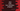# Write a love calculator program in Java# Java love calculator program :

In this tutorial, we will learn how to create one love calculator program. This program will take two names as inputs, calculates the percentage and prints it out. How the program is calculating the percentage, I am explaining below.

You can change the algorithm to any other way to calculate the percentage.

## Algorithm :

The below algorithm we are going to use in this program :

1. Get the first and the second names as input from the user.
2. Convert both to lowercase.
3. Find the sum of all ASCII values of the characters for both names.
4. Similarly, find the sum of all ASCII values of the characters for ‘love’.
5. Add the sum of both values calculated on step 3.
6. Find the sum of all digits of both result from step 4 and step 5.
7. If the sum for names is greater than sum for love, subtract the difference amount from sum of love and assign it as the sum of names.
8. Calculate the percentage as _(sum of names/sum of loves) * 100 _.

## Example :

Let’s say, we are calculating for Alex and Liza

1. Convert both to lowercase : alex and liza.
2. Find the sum of ASCII of each character :
``````* For _alex_, it is : _97 +108 +101 +120 = 426_

* For _liza_, it is : _108 +105 +122 +97 = 432_.``````
1. Find the sum of ASCII of love : 108 +111 +118 +101 = 438.
2. Add the sum of step 3 : 426 + 432 = 858.
3. Find the sum of all digits : for names it is 8 + 5 + 8 = 21. For ‘love’, it is 4 + 3 + 8 = 15
4. sum of names(22) > sum of love(15). So, new value of sum of names is 15 - (21-15) = 9
5. Percentage : (9/15) * 100 = 60%

## Java Program :

``````import java.util.Scanner;

public class LoveCalculator {

private static int findSum(int no) {
int sum = 0;
while (no > 0) {
sum += no % 10;
no /= 10;
}
return sum;
}

public static void main(String[] args) {
Scanner sc = new Scanner(System.in);

final String LOVE = "love";

String firstName;
String secondName;

int firstSum;
int secondSum;
int loveSum;
int totalSum;

while (true) {
System.out.println("♥️♥♥️♥♥️♥♥️♥♥️♥♥️♥♥️♥♥️♥♥️♥♥️♥♥️♥♥️♥♥️️");
System.out.println("Enter q to exit : ");
System.out.println("First Name : ");
firstName = sc.nextLine();

if (firstName.equals("q")) {
break;
}
System.out.println("Second Name : ");
secondName = sc.nextLine();

firstSum = 0;
secondSum = 0;
loveSum = 0;

firstName = firstName.toLowerCase();
secondName = secondName.toLowerCase();

for (var i = 0; i < firstName.length(); i++) {
firstSum += firstName.charAt(i);
}

for (var i = 0; i < secondName.length(); i++) {
secondSum += secondName.charAt(i);
}

for (var i = 0; i < LOVE.length(); i++) { loveSum += LOVE.charAt(i); } totalSum = findSum(firstSum + secondSum); loveSum = findSum(loveSum); if (totalSum > loveSum) {
totalSum = loveSum - (totalSum - loveSum);
}

System.out.println("Love % : " + (totalSum * 100 / loveSum));

}

}

}``````

### Sample Output :

``````♥️♥♥️♥♥️♥♥️♥♥️♥♥️♥♥️♥♥️♥♥️♥♥️♥♥️♥♥️♥♥️️
Enter q to exit :
First Name :
Alex
Second Name :
Liza
Love % : 60
♥️♥♥️♥♥️♥♥️♥♥️♥♥️♥♥️♥♥️♥♥️♥♥️♥♥️♥♥️♥♥️️
Enter q to exit :
First Name :
Bob
Second Name :
Liza
Love % : 73``````

The program will exit only if the user enters q as the first name.